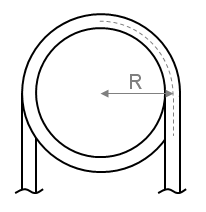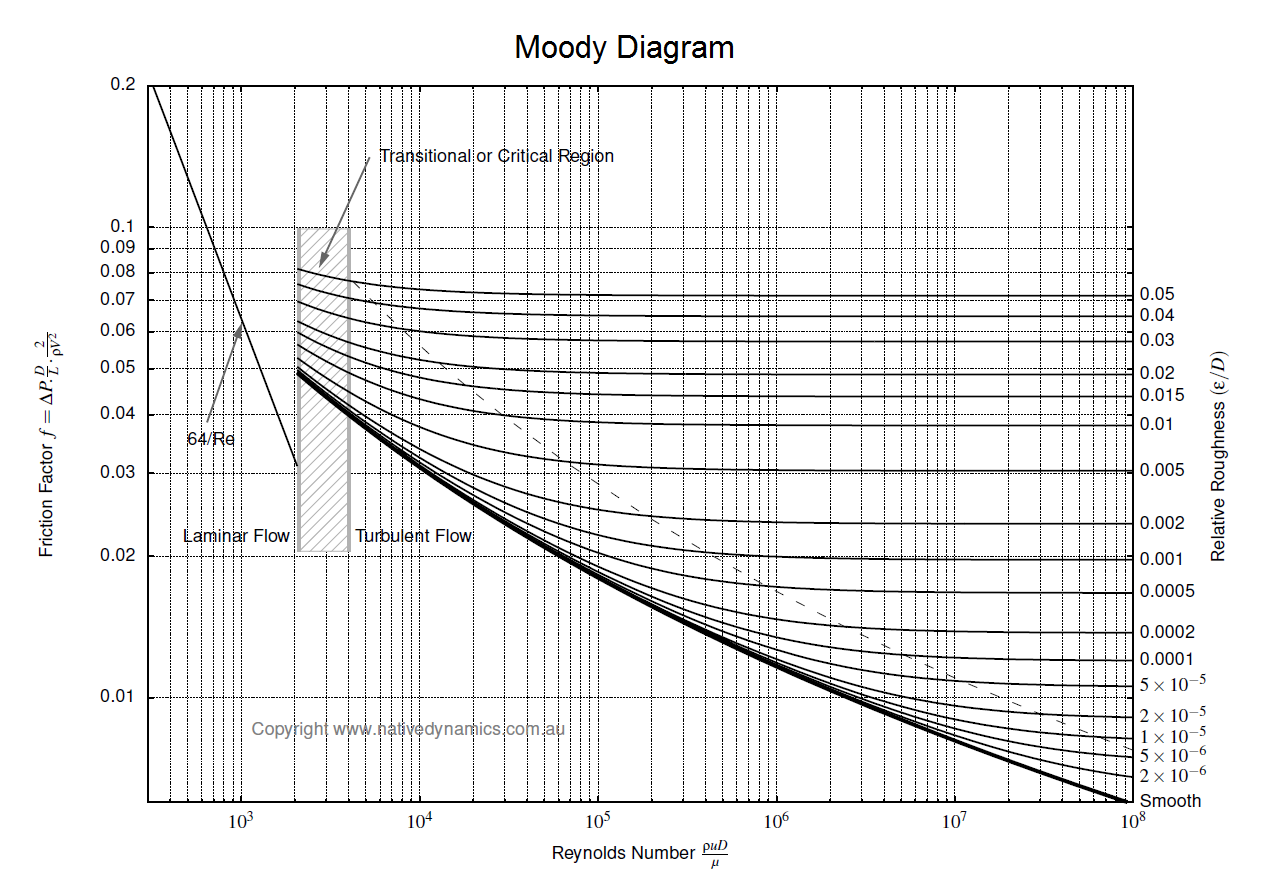f
Neutrium

# Pipe Diameter

Nominal Pipe Characteristics in Metric Units

Nominal Pipe Size (NPS) is a defined set of standard pipe sizes commonly used for process piping. NPS piping is defined in terms of a nominal diameter and wall thickness (defined by the pipe schedule). This article provides characteristics for NPS piping in metric units.

Nominal Pipe Characteristics in Imperial Units

Nominal Pipe Size (NPS) is a defined set of standard pipe sizes commonly used for process piping. NPS piping is defined in terms of a nominal diameter and wall thickness (defined by the pipe schedule). This article provides characteristics for NPS piping in Imperial units.

Pressure Loss from Fittings in Pipe Summary

Fittings such as elbows, tees, valves and reducers represent a significant component of the pressure loss in most pipe systems. This article discusses the differences between several popular methods for determining the pressure loss through fittings. The methods discussed for fittings are: the equivalent length method, the K method (velocity head method or resistance coefficient method), the two-K method and the three-K method. In this article we also discuss method for calculating pressure loss through pipe size changes as well as control valves.

Friction Factor for Flow in Coils and Curved PipeIn order to determine the pressure drop in a pipe or coil the friction factor must first be calculated. This article presents the equations which may be used to determine the friction factor in coils and curved pipe.

Pressure Loss in PipeTo determine the pressure loss or flow rate through pipe knowledge of the friction between the fluid and the pipe is required. This article describes how to incorporate friction into pressure loss or fluid flow calculations. It also outlines several methods for determining the Darcy friction factor for rough and smooth pipes in both the turbulent and laminar flow regime. Finally this article discusses which correlation for pressure loss in pipe is the most appropriate.